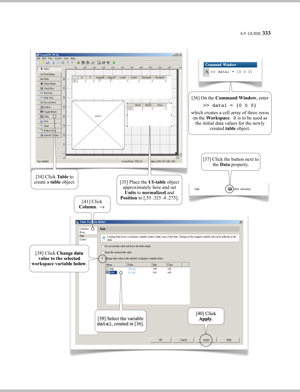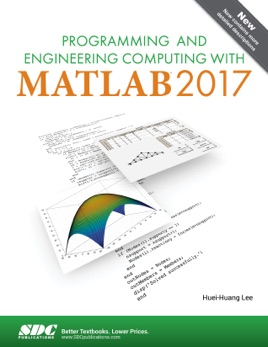• 84,99 zł

## Publisher Description

• Designed for engineering students completely new to programming with MATLAB
• Uses a learn by doing approach with step by step instructions
• Contains line by line descriptions of code presented in the book
• Case studies and examples are used throughout the book
• Devotes an entire chapter to designing graphical user interfaces
• Now covers the App Designer and the Live Script Editor

This book is designed for undergraduate students completely new to programming with MATLAB. Case studies and examples are used extensively throughout this book and are at the core of what makes this book so unique. The author believes that the best way to learn MATLAB is to study programs written by experienced programmers and that the quality of these example programs determines the quality of the book. The examples in this book are carefully designed to teach you MATLAB programming as well as to inspire within you your own problem solving potential. Most of the examples used in this book are designed to solve a whole class of problems, rather than a single, specific problem.

A learn by doing teaching approach is used all through the book. You are guided to tackle a problem using MATLAB commands first and then the commands are explained line by line. This process of learning through hands on experience is one of the most efficient and pain-free ways of learning MATLAB. This approach, together with the extensive use of ordered textboxes, figures, and tables, greatly reduces the size of the book, while still providing you with a book that’s comprehensive and easy to follow.

The first chapter of this book introduces the MATLAB programming environment and familiarizes you with MATLAB’s core functionality. Chapters two through nine discuss basic MATLAB functionalities in a progressive and comprehensive way. The chapters start out simple and build in complexity as you advance through the book. Chapters ten through thirteen cover advanced topics that are particularly useful in college programs. Each chapter consists of sections, each covering a topic and providing one or more examples. Related MATLAB functions are organized at the end of a section. Additional exercise problems are provided at the end of chapters two through nine.

Examples in each section are presented in a consistent way. An example is usually described first, followed by a MATLAB script. Any resulting text and graphics output (and in some cases inputs) that are produced from running a script are presented and discussed. Finally, the remainder of each section is devoted to explaining the purpose of the lines of the script.

Who this book is for

This book is developed mainly for undergraduate engineering students. It may be used in courses such as Computers in Engineering, or others that use MATLAB as a software platform. It can also be used as a self-study book for learning MATLAB.

College level engineering examples are used in this book. Background knowledge for these engineering examples is illustrated as thoroughly as possible.

New in this edition

This edition now covers the MATLAB App Designer and Live Script Editor. Also, all the descriptions in the book have been greatly expanded.

1.   Getting Started, Desktop Environment, and Overview
2.   Data Types, Operators, and Expressions
3.   Flow Controls, Functions, and Programs
4.   Cell Arrays, Structures, Tables, and User-Defined Classes
5.   Data Visualization: Plots
6.   Animations, Images, Audios, and Videos
7.   Data Import and Export
8.   Graphical User Interfaces
9.   Symbolic Mathematics
10. Linear Algebra, Polynomial, Curve Fitting, and Interpolation
11. Differentiation, Integration, and Differential Equations
12. Systems of Nonlinear Equations and Optimization
13. Statistics

•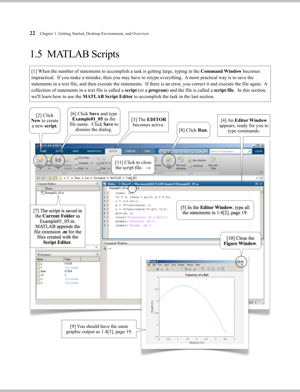•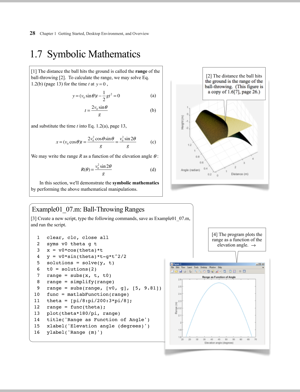•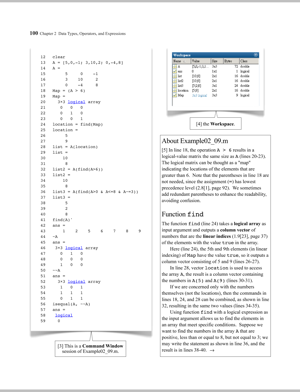•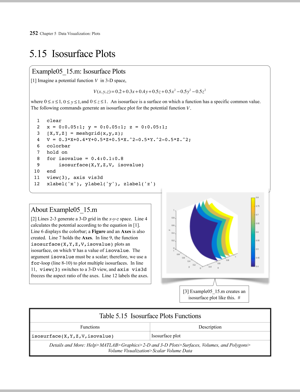•